# 3.7 Rational functions  (Page 11/16)

 Page 11 / 16

$f\left(x\right)=\frac{x}{2x+1}$

Local behavior: $\text{\hspace{0.17em}}x\to -{\frac{1}{2}}^{+},f\left(x\right)\to -\infty ,x\to -{\frac{1}{2}}^{-},f\left(x\right)\to \infty \text{\hspace{0.17em}}$

End behavior: $\text{\hspace{0.17em}}x\to ±\infty ,f\left(x\right)\to \frac{1}{2}$

$f\left(x\right)=\frac{2x}{x-6}$

$f\left(x\right)=\frac{-2x}{x-6}$

Local behavior: $\text{\hspace{0.17em}}x\to {6}^{+},f\left(x\right)\to -\infty ,x\to {6}^{-},f\left(x\right)\to \infty ,\text{\hspace{0.17em}}$ End behavior: $\text{\hspace{0.17em}}x\to ±\infty ,f\left(x\right)\to -2$

$f\left(x\right)=\frac{{x}^{2}-4x+3}{{x}^{2}-4x-5}$

$f\left(x\right)=\frac{2{x}^{2}-32}{6{x}^{2}+13x-5}$

Local behavior: $\text{\hspace{0.17em}}x\to -{\frac{1}{3}}^{+},f\left(x\right)\to \infty ,x\to -{\frac{1}{3}}^{-},\text{\hspace{0.17em}}$ $f\left(x\right)\to -\infty ,x\to {\frac{5}{2}}^{-},f\left(x\right)\to \infty ,x\to {\frac{5}{2}}^{+}$ , $f\left(x\right)\to -\infty$

End behavior: $x\to ±\infty ,$ $f\left(x\right)\to \frac{1}{3}$

For the following exercises, find the slant asymptote of the functions.

$f\left(x\right)=\frac{24{x}^{2}+6x}{2x+1}$

$f\left(x\right)=\frac{4{x}^{2}-10}{2x-4}$

$y=2x+4$

$f\left(x\right)=\frac{81{x}^{2}-18}{3x-2}$

$f\left(x\right)=\frac{6{x}^{3}-5x}{3{x}^{2}+4}$

$y=2x$

$f\left(x\right)=\frac{{x}^{2}+5x+4}{x-1}$

## Graphical

For the following exercises, use the given transformation to graph the function. Note the vertical and horizontal asymptotes.

The reciprocal function shifted up two units.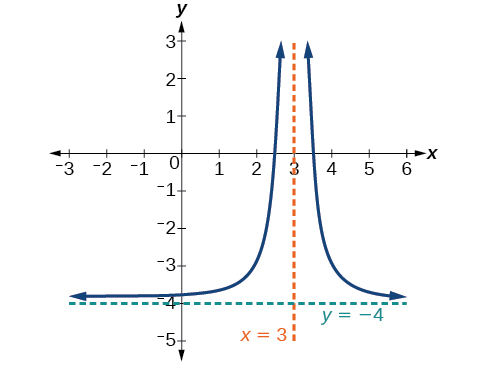The reciprocal function shifted down one unit and left three units.

The reciprocal squared function shifted to the right 2 units.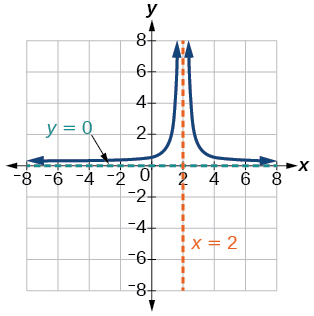The reciprocal squared function shifted down 2 units and right 1 unit.

For the following exercises, find the horizontal intercepts, the vertical intercept, the vertical asymptotes, and the horizontal or slant asymptote of the functions. Use that information to sketch a graph.

$p\left(x\right)=\frac{2x-3}{x+4}$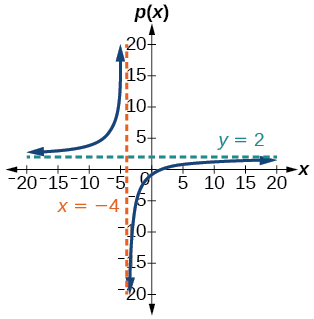$q\left(x\right)=\frac{x-5}{3x-1}$

$s\left(x\right)=\frac{4}{{\left(x-2\right)}^{2}}$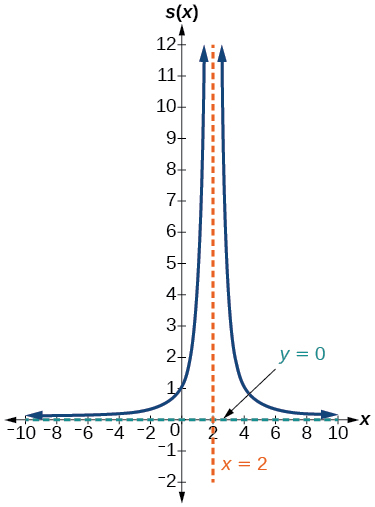$r\left(x\right)=\frac{5}{{\left(x+1\right)}^{2}}$

$f\left(x\right)=\frac{3{x}^{2}-14x-5}{3{x}^{2}+8x-16}$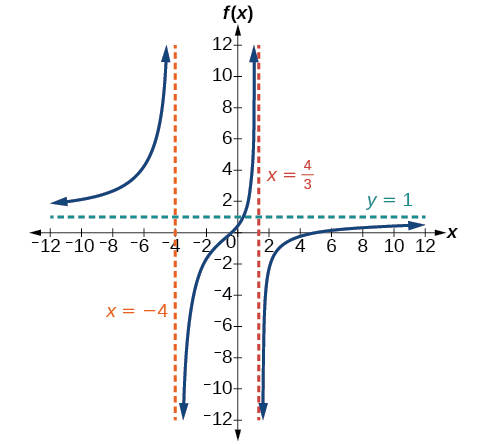$g\left(x\right)=\frac{2{x}^{2}+7x-15}{3{x}^{2}-14+15}$

$a\left(x\right)=\frac{{x}^{2}+2x-3}{{x}^{2}-1}$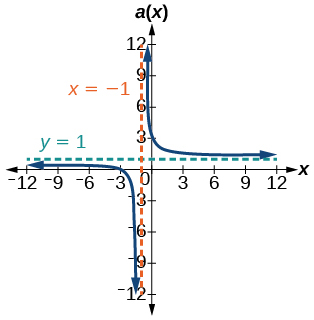$b\left(x\right)=\frac{{x}^{2}-x-6}{{x}^{2}-4}$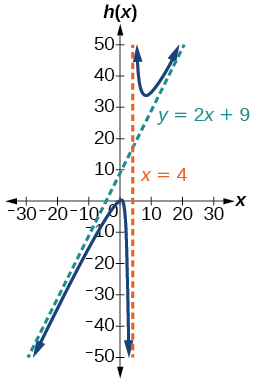$k\left(x\right)=\frac{2{x}^{2}-3x-20}{x-5}$

$w\left(x\right)=\frac{\left(x-1\right)\left(x+3\right)\left(x-5\right)}{{\left(x+2\right)}^{2}\left(x-4\right)}$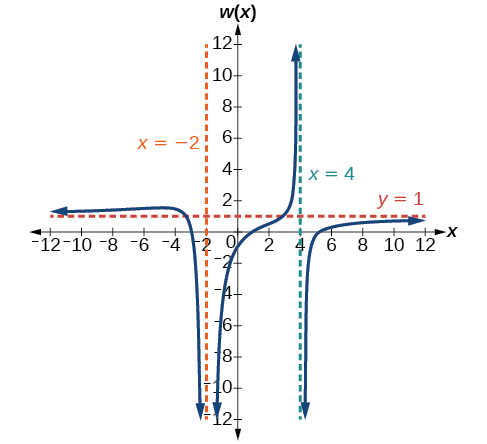$z\left(x\right)=\frac{{\left(x+2\right)}^{2}\left(x-5\right)}{\left(x-3\right)\left(x+1\right)\left(x+4\right)}$

For the following exercises, write an equation for a rational function with the given characteristics.

Vertical asymptotes at $\text{\hspace{0.17em}}x=5\text{\hspace{0.17em}}$ and $\text{\hspace{0.17em}}x=-5,\text{\hspace{0.17em}}$ x -intercepts at $\text{\hspace{0.17em}}\left(2,0\right)\text{\hspace{0.17em}}$ and $\text{\hspace{0.17em}}\left(-1,0\right),\text{\hspace{0.17em}}$ y -intercept at $\text{\hspace{0.17em}}\left(0,4\right)$

$y=50\frac{{x}^{2}-x-2}{{x}^{2}-25}$

Vertical asymptotes at $\text{\hspace{0.17em}}x=-4\text{\hspace{0.17em}}$ and $\text{\hspace{0.17em}}x=-1,\text{\hspace{0.17em}}$ x- intercepts at $\text{\hspace{0.17em}}\left(1,0\right)\text{\hspace{0.17em}}$ and $\text{\hspace{0.17em}}\left(5,0\right),\text{\hspace{0.17em}}$ y- intercept at $\text{\hspace{0.17em}}\left(0,7\right)$

Vertical asymptotes at $\text{\hspace{0.17em}}x=-4\text{\hspace{0.17em}}$ and $\text{\hspace{0.17em}}x=-5,\text{\hspace{0.17em}}$ x -intercepts at $\text{\hspace{0.17em}}\left(4,0\right)\text{\hspace{0.17em}}$ and $\text{\hspace{0.17em}}\left(-6,0\right),\text{\hspace{0.17em}}$ Horizontal asymptote at $\text{\hspace{0.17em}}y=7$

$y=7\frac{{x}^{2}+2x-24}{{x}^{2}+9x+20}$

Vertical asymptotes at $\text{\hspace{0.17em}}x=-3\text{\hspace{0.17em}}$ and $\text{\hspace{0.17em}}x=6,\text{\hspace{0.17em}}$ x -intercepts at $\text{\hspace{0.17em}}\left(-2,0\right)\text{\hspace{0.17em}}$ and $\text{\hspace{0.17em}}\left(1,0\right),\text{\hspace{0.17em}}$ Horizontal asymptote at $\text{\hspace{0.17em}}y=-2$

Vertical asymptote at $\text{\hspace{0.17em}}x=-1,\text{\hspace{0.17em}}$ Double zero at $\text{\hspace{0.17em}}x=2,\text{\hspace{0.17em}}$ y -intercept at $\text{\hspace{0.17em}}\left(0,2\right)$

$y=\frac{1}{2}\frac{{x}^{2}-4x+4}{x+1}$

Vertical asymptote at $\text{\hspace{0.17em}}x=3,\text{\hspace{0.17em}}$ Double zero at $\text{\hspace{0.17em}}x=1,\text{\hspace{0.17em}}$ y -intercept at $\text{\hspace{0.17em}}\left(0,4\right)$

For the following exercises, use the graphs to write an equation for the function.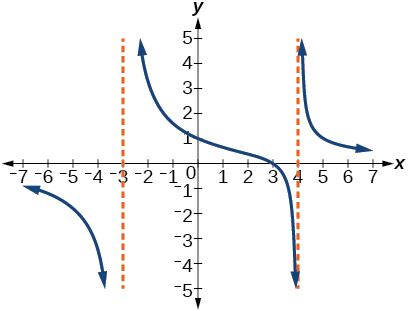$y=4\frac{x-3}{{x}^{2}-x-12}$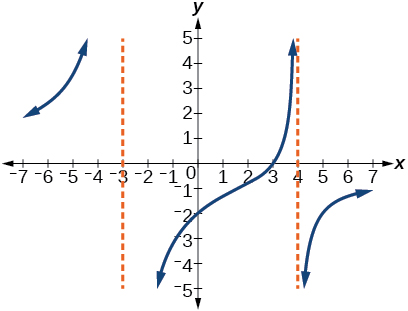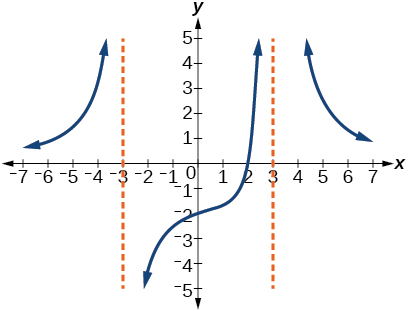$y=-9\frac{x-2}{{x}^{2}-9}$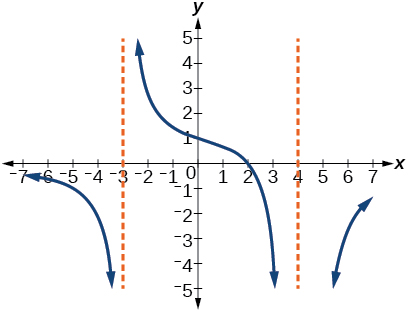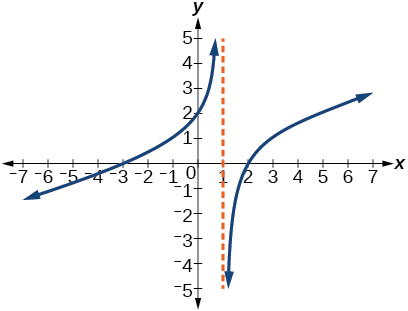$y=\frac{1}{3}\frac{{x}^{2}+x-6}{x-1}$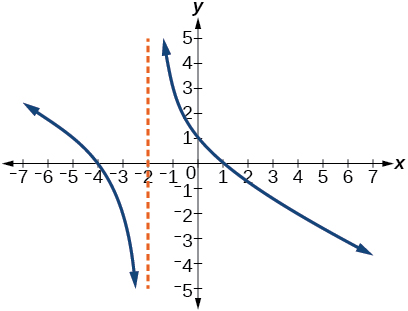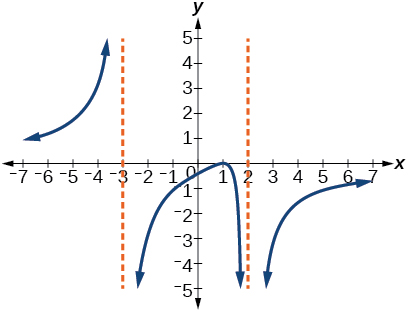$y=-6\frac{{\left(x-1\right)}^{2}}{\left(x+3\right){\left(x-2\right)}^{2}}$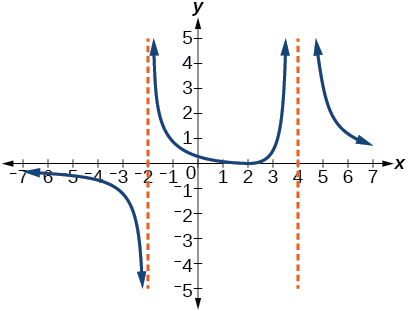## Numeric

For the following exercises, make tables to show the behavior of the function near the vertical asymptote and reflecting the horizontal asymptote

$f\left(x\right)=\frac{1}{x-2}$

 $x$ 2.01 2.001 2.0001 1.99 1.999 $y$ 100 1,000 10,000 –100 –1,000
$x$ 10 100 1,000 10,000 100,000
$y$ .125 .0102 .001 .0001 .00001

Vertical asymptote $\text{\hspace{0.17em}}x=2,\text{\hspace{0.17em}}$ Horizontal asymptote $\text{\hspace{0.17em}}y=0$

$f\left(x\right)=\frac{x}{x-3}$

$f\left(x\right)=\frac{2x}{x+4}$

 $x$ –4.1 –4.01 –4.001 –3.99 –3.999 $y$ 82 802 8,002 –798 –7998
 $x$ 10 100 1,000 10,000 100,000 $y$ 1.4286 1.9331 1.992 1.9992 1.999992

Vertical asymptote $\text{\hspace{0.17em}}x=-4,\text{\hspace{0.17em}}$ Horizontal asymptote $\text{\hspace{0.17em}}y=2$

$f\left(x\right)=\frac{2x}{{\left(x-3\right)}^{2}}$

$f\left(x\right)=\frac{{x}^{2}}{{x}^{2}+2x+1}$

 $x$ –.9 –.99 –.999 –1.1 –1.01 $y$ 81 9,801 998,001 121 10,201
 $x$ 10 100 1,000 10,000 100,000 $y$ 0.82645 0.9803 .998 .9998

Vertical asymptote $\text{\hspace{0.17em}}x=-1,\text{\hspace{0.17em}}$ Horizontal asymptote $\text{\hspace{0.17em}}y=1$

## Technology

For the following exercises, use a calculator to graph $\text{\hspace{0.17em}}f\left(x\right).\text{\hspace{0.17em}}$ Use the graph to solve $\text{\hspace{0.17em}}f\left(x\right)>0.$

$f\left(x\right)=\frac{2}{x+1}$

$f\left(x\right)=\frac{4}{2x-3}$

$\left(\frac{3}{2},\infty \right)$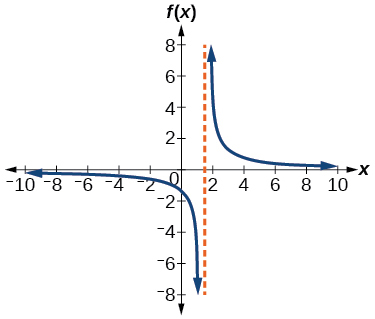$f\left(x\right)=\frac{2}{\left(x-1\right)\left(x+2\right)}$

$f\left(x\right)=\frac{x+2}{\left(x-1\right)\left(x-4\right)}$

$\left(-2,1\right)\cup \left(4,\infty \right)$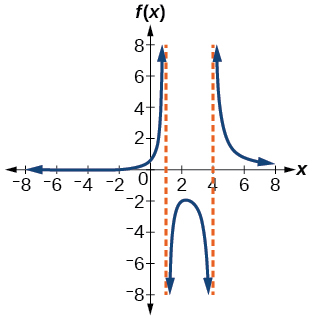$f\left(x\right)=\frac{{\left(x+3\right)}^{2}}{{\left(x-1\right)}^{2}\left(x+1\right)}$

## Extensions

For the following exercises, identify the removable discontinuity.

$f\left(x\right)=\frac{{x}^{2}-4}{x-2}$

$\left(2,4\right)$

$f\left(x\right)=\frac{{x}^{3}+1}{x+1}$

$f\left(x\right)=\frac{{x}^{2}+x-6}{x-2}$

$\left(2,5\right)$

$f\left(x\right)=\frac{2{x}^{2}+5x-3}{x+3}$

$f\left(x\right)=\frac{{x}^{3}+{x}^{2}}{x+1}$

$\left(–1,\text{1}\right)$

## Real-world applications

For the following exercises, express a rational function that describes the situation.

A large mixing tank currently contains 200 gallons of water, into which 10 pounds of sugar have been mixed. A tap will open, pouring 10 gallons of water per minute into the tank at the same time sugar is poured into the tank at a rate of 3 pounds per minute. Find the concentration (pounds per gallon) of sugar in the tank after $\text{\hspace{0.17em}}t\text{\hspace{0.17em}}$ minutes.

A large mixing tank currently contains 300 gallons of water, into which 8 pounds of sugar have been mixed. A tap will open, pouring 20 gallons of water per minute into the tank at the same time sugar is poured into the tank at a rate of 2 pounds per minute. Find the concentration (pounds per gallon) of sugar in the tank after $\text{\hspace{0.17em}}t\text{\hspace{0.17em}}$ minutes.

$C\left(t\right)=\frac{8+2t}{300+20t}$

For the following exercises, use the given rational function to answer the question.

The concentration $\text{\hspace{0.17em}}C\text{\hspace{0.17em}}$ of a drug in a patient’s bloodstream $\text{\hspace{0.17em}}t\text{\hspace{0.17em}}$ hours after injection in given by $\text{\hspace{0.17em}}C\left(t\right)=\frac{2t}{3+{t}^{2}}.\text{\hspace{0.17em}}$ What happens to the concentration of the drug as $\text{\hspace{0.17em}}t\text{\hspace{0.17em}}$ increases?

The concentration $\text{\hspace{0.17em}}C\text{\hspace{0.17em}}$ of a drug in a patient’s bloodstream $\text{\hspace{0.17em}}t\text{\hspace{0.17em}}$ hours after injection is given by $\text{\hspace{0.17em}}C\left(t\right)=\frac{100t}{2{t}^{2}+75}.\text{\hspace{0.17em}}$ Use a calculator to approximate the time when the concentration is highest.

For the following exercises, construct a rational function that will help solve the problem. Then, use a calculator to answer the question.

An open box with a square base is to have a volume of 108 cubic inches. Find the dimensions of the box that will have minimum surface area. Let $\text{\hspace{0.17em}}x\text{\hspace{0.17em}}$ = length of the side of the base.

A rectangular box with a square base is to have a volume of 20 cubic feet. The material for the base costs 30 cents/ square foot. The material for the sides costs 10 cents/square foot. The material for the top costs 20 cents/square foot. Determine the dimensions that will yield minimum cost. Let $\text{\hspace{0.17em}}x\text{\hspace{0.17em}}$ = length of the side of the base.

$A\left(x\right)=50{x}^{2}+\frac{800}{x}.\text{\hspace{0.17em}}$ 2 by 2 by 5 feet.

A right circular cylinder has volume of 100 cubic inches. Find the radius and height that will yield minimum surface area. Let $\text{\hspace{0.17em}}x\text{\hspace{0.17em}}$ = radius.

A right circular cylinder with no top has a volume of 50 cubic meters. Find the radius that will yield minimum surface area. Let $\text{\hspace{0.17em}}x\text{\hspace{0.17em}}$ = radius.

$A\left(x\right)=\pi {x}^{2}+\frac{100}{x}.\text{\hspace{0.17em}}$ Radius = 2.52 meters.

A right circular cylinder is to have a volume of 40 cubic inches. It costs 4 cents/square inch to construct the top and bottom and 1 cent/square inch to construct the rest of the cylinder. Find the radius to yield minimum cost. Let $\text{\hspace{0.17em}}x\text{\hspace{0.17em}}$ = radius.

can you not take the square root of a negative number
No because a negative times a negative is a positive. No matter what you do you can never multiply the same number by itself and end with a negative
lurverkitten
Actually you can. you get what's called an Imaginary number denoted by i which is represented on the complex plane. The reply above would be correct if we were still confined to the "real" number line.
Liam
Suppose P= {-3,1,3} Q={-3,-2-1} and R= {-2,2,3}.what is the intersection
can I get some pretty basic questions
In what way does set notation relate to function notation
Ama
is precalculus needed to take caculus
It depends on what you already know. Just test yourself with some precalculus questions. If you find them easy, you're good to go.
Spiro
the solution doesn't seem right for this problem
what is the domain of f(x)=x-4/x^2-2x-15 then
x is different from -5&3
Seid
All real x except 5 and - 3
Spiro
***youtu.be/ESxOXfh2Poc
Loree
how to prroved cos⁴x-sin⁴x= cos²x-sin²x are equal
Don't think that you can.
Elliott
By using some imaginary no.
Tanmay
how do you provided cos⁴x-sin⁴x = cos²x-sin²x are equal
What are the question marks for?
Elliott
Someone should please solve it for me Add 2over ×+3 +y-4 over 5 simplify (×+a)with square root of two -×root 2 all over a multiply 1over ×-y{(×-y)(×+y)} over ×y
For the first question, I got (3y-2)/15 Second one, I got Root 2 Third one, I got 1/(y to the fourth power) I dont if it's right cause I can barely understand the question.
Is under distribute property, inverse function, algebra and addition and multiplication function; so is a combined question
Abena
find the equation of the line if m=3, and b=-2
graph the following linear equation using intercepts method. 2x+y=4
Ashley
how
Wargod
what?
John
ok, one moment
UriEl
how do I post your graph for you?
UriEl
it won't let me send an image?
UriEl
also for the first one... y=mx+b so.... y=3x-2
UriEl
y=mx+b you were already given the 'm' and 'b'. so.. y=3x-2
Tommy
Please were did you get y=mx+b from
Abena
y=mx+b is the formula of a straight line. where m = the slope & b = where the line crosses the y-axis. In this case, being that the "m" and "b", are given, all you have to do is plug them into the formula to complete the equation.
Tommy
thanks Tommy
Nimo
0=3x-2 2=3x x=3/2 then . y=3/2X-2 I think
Given
co ordinates for x x=0,(-2,0) x=1,(1,1) x=2,(2,4)
neil
"7"has an open circle and "10"has a filled in circle who can I have a set builder notation
Where do the rays point?
Spiro
x=-b+_Гb2-(4ac) ______________ 2a
I've run into this: x = r*cos(angle1 + angle2) Which expands to: x = r(cos(angle1)*cos(angle2) - sin(angle1)*sin(angle2)) The r value confuses me here, because distributing it makes: (r*cos(angle2))(cos(angle1) - (r*sin(angle2))(sin(angle1)) How does this make sense? Why does the r distribute once
so good
abdikarin
this is an identity when 2 adding two angles within a cosine. it's called the cosine sum formula. there is also a different formula when cosine has an angle minus another angle it's called the sum and difference formulas and they are under any list of trig identities
strategies to form the general term
carlmark
consider r(a+b) = ra + rb. The a and b are the trig identity.
Mike
How can you tell what type of parent function a graph is ?
generally by how the graph looks and understanding what the base parent functions look like and perform on a graph
William
if you have a graphed line, you can have an idea by how the directions of the line turns, i.e. negative, positive, zero
William
y=x will obviously be a straight line with a zero slope
William
y=x^2 will have a parabolic line opening to positive infinity on both sides of the y axis vice versa with y=-x^2 you'll have both ends of the parabolic line pointing downward heading to negative infinity on both sides of the y axis
William
y=x will be a straight line, but it will have a slope of one. Remember, if y=1 then x=1, so for every unit you rise you move over positively one unit. To get a straight line with a slope of 0, set y=1 or any integer.
Aaron
yes, correction on my end, I meant slope of 1 instead of slope of 0
William
what is f(x)=
I don't understand
Joe
Typically a function 'f' will take 'x' as input, and produce 'y' as output. As 'f(x)=y'. According to Google, "The range of a function is the complete set of all possible resulting values of the dependent variable (y, usually), after we have substituted the domain."
Thomas
Sorry, I don't know where the "Â"s came from. They shouldn't be there. Just ignore them. :-)
Thomas
Darius
Thanks.
Thomas
Â
Thomas
It is the Â that should not be there. It doesn't seem to show if encloses in quotation marks. "Â" or 'Â' ... Â
Thomas
Now it shows, go figure?
ThomasByBy OpenStaxBy Janet ForresterBy Madison ChristianBy OpenStaxBy OpenStaxBy OpenStaxBy Brooke DelaneyBy Abishek DevarajBy OpenStaxBy